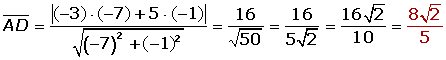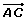### Scalar Projection### Vector Projection

The vector projection is the unit vector ofby the scalar projection of u on v.The scalar projection of u on v is the magnitude of the vector projection of u on v.

Calculate the vector projection of= (2, 1) on the vector= (−3, 4).Calculate the vector projection ofon the vector.Calculate the scalar projection of the vectoron the vectorif: A = (6,0), B = (3,5) and C = (−1,−1).If the vertices of a triangle are A = (6, 0), B = (3, 5) and C = (−1, −1), compute the scalar projections of the sides AB and CB on AC, and check that their sum is equal to the length of AC.= (-3, 5)= (3, -5)= (-7, -1)= (7, 1)= (-4, -6)= (4, 6)·= |(−3)· (−7) + 5 · (-1)| = 16·= 7· 4 + 1 · 6 = 34Did you like the article?(1 votes, average: 5.00 out of 5)Loading...

Emma

I am passionate about travelling and currently live and work in Paris. I like to spend my time reading, gardening, running, learning languages and exploring new places.

Did you like
this resource?

Bravo!